Next: Moving windows for nonstationarity Up: DIP PICKING WITHOUT DIP Previous: DIP PICKING WITHOUT DIP

## The plane-wave destructor

A plane wave in a wave field u(t,x)=u(t-px) with stepout p can be extinguished with a partial differential operator, which we write as a matrix, where(25) (26)
The parameter p is called the wavenumber" or Snell parameter," and |p| can take on any value less than 1/v, where v is the medium velocity. The angle of propagation of the wave is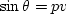.

We need a method of discretization that allows the mesh for du/dt to overlay exactly du/dx. To this end I chose to represent the t-derivative by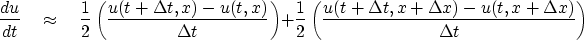(27)
and the x-derivative by an analogous expression with t and x interchanged. Now the difference operator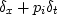is a two-dimensional filter that fits on adifferencing star. As a matrix operation, this two-dimensional convolution is denoted.(More details about finite differencing can be found in IEI.)

The program wavekill1() applies the operator,which can be specialized to the operators,,.

#   vv = (aa Dx + pp Dt) uu
#
subroutine wavekill1( aa, pp,    n1,n2,uu,  vv )
integer i1,i2,                      n1,n2
real aa, pp, s11, s12, s21, s22, uu(n1,n2), vv( n1-1,  n2-1)
s11 = -aa-pp;   s12 = aa-pp
s21 = -aa+pp;   s22 = aa+pp
call null(                                  vv,(n1-1)*(n2-1))
do i1= 1, n1-1 {                # vv is one point shorter than uu on both axes.
do i2= 1, n2-1 {
vv(i1,i2) = vv(i1,i2) +
uu(i1  ,i2) * s11 + uu(i1  ,i2+1) * s12 +
uu(i1+1,i2) * s21 + uu(i1+1,i2+1) * s22
}}
return; end

I carefully arranged the side boundaries so that the filter never runs off the sides of the data. Thus the output is shorter than the input by one point on both the t-axis and the x-axis. The reason for using these side boundaries is that large datasets can be chopped into independent sections without the boundaries themselves affecting the result. By chopping a large dataset into sections, we can handle curved events as piecewise linear.

When only one wave is present and the data is adequately sampled, then finding the best value of p is a single-parameter, linear least-squares problem. Letbe an abstract vector whose components are values of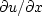taken on a mesh in (t,x). Likewise, letcontain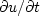.Since we want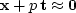,we minimize the quadratic function of p,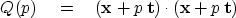(28)
by setting to zero the derivative. We get(29)
Since data will not always fit the model very well, it may be helpful to have some way to measure how good the fit is. I suggest(30)
which, on inserting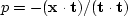,leads to C, where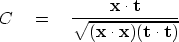(31)
is known as the normalized correlation.''  The program for this calculation is straightforward. I named the program puck() to denote picking on a continuum.

# measure coherency and dip, and compute residual  res = (Dx + p Dt) uu
#
subroutine puck ( n1, n2, uu, coh, pp, res )
integer i1, i2,   n1, n2
real    uu(n1,n2), res(n1,n2), xx, xt, tt, coh, pp
temporary real dx(n1,n2-1), dt(n1-1,n2-1)
call wavekill1( 1., 0.,  n1,n2 , uu, dx)
call wavekill1( 0., 1.,  n1,n2 , uu, dt)
xx = 1.e-30; tt = 1.e-30; xt = 0.
do i1= 1, n1-1 {
do i2= 1, n2-1 {
xt = xt + dt(i1,i2) * dx(i1,i2)
tt = tt + dt(i1,i2) * dt(i1,i2)
xx = xx + dx(i1,i2) * dx(i1,i2)
}}
coh = sqrt((xt/tt)*(xt/xx))
pp  =  - xt/tt
call wavekill1( 1., pp,  n1,n2 , uu, res)
return; end


Finally and parenthetically, an undesirable feature of the plane-wave destructor method is that the residualhas no particular relation to the data,unlike in time-series analysis--see chapter. Another disadvantage, well known to people who routinely work with finite-difference solutions to partial differential equations, is that for short wavelengths a difference operator is not the same as a differential operator; thereby the numerical value of p is biased.Next: Moving windows for nonstationarity Up: DIP PICKING WITHOUT DIP Previous: DIP PICKING WITHOUT DIP
Stanford Exploration Project
10/21/1998